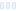# Basic Four

## General Mills

Main info:

Basic Four
General Mills
1 serving
200.0 Calories
44 g
2.0 g
4.0 g

4.0 g
0 mg
1.0 g
280.0 mg
0 g
0 g

Percent calories from...
Nutrition Facts
For a Serving Size of (g)
How many calories are in Basic Four? Amount of calories in Basic Four: Calories Calories from Fat (%)
% Daily Value *
How much fat is in Basic Four? Amount of fat in Basic Four: Total Fat
How much saturated fat is in Basic Four? Amount of saturated fat in Basic Four: Saturated fat
How much monounsaturated fat is in Basic Four? Amount of monounsaturated fat in Basic Four: Monounsaturated fat
How much polyunsaturated fat is in Basic Four? Amount of polyunsaturated fat in Basic Four: Polyunsaturated fat
How much sodium is in Basic Four? Amount of sodium in Basic Four: Sodium
How much potassium is in Basic Four? Amount of potassium in Basic Four: Potassium
How many carbs are in Basic Four? Amount of carbs in Basic Four: Carbohydrates
How many net carbs are in Basic Four? Amount of net carbs in Basic Four: Net carbs
How much fiber is in Basic Four? Amount of fiber in Basic Four: Fiber
How much glucose is in Basic Four? Amount of glucose in Basic Four: Glucose
How much protein is in Basic Four? Amount of protein in Basic Four: Protein
Vitamins and minerals
How much Vitamin A is in Basic Four? Amount of Vitamin A in Basic Four: Vitamin A
How much Vitamin C is in Basic Four? Amount of Vitamin C in Basic Four: Vitamin C
How much Calcium is in Basic Four? Amount of Calcium in Basic Four: Calcium
How much Iron is in Basic Four? Amount of Iron in Basic Four: Iron
Fatty acids
Amino acids
* The Percent Daily Values are based on a 2,000 calorie diet, so your values may change depending on your calorie needs.Loading similar foods...
Note: Any items purchased after clicking our Amazon buttons will give us a little referral bonus. If you do click them, thank you!Be cool

- Zen orangeI never skip arm day

- Buff broccoli